# Metal Tutorial with Swift 3 Part 2: Moving to 3D

In this second part of our Metal tutorial series, learn how to create a rotating 3D cube using Apple’s built-in 3D graphics API.

Update: This tutorial has been updated for Xcode 8.2 and Swift 3.

Welcome back to our iOS Metal tutorial series!

In the first part of the series, you learned how to get started with Metal and render a simple 2D triangle.

In this second part of the series, you’ll learn how to set up a series of matrix transformations to move to full 3D. In the process, you will learn:

• How to use model, view, and projection transformations
• How to use matrices to transform geometry
• How to use backface culling to optimize your drawing

Get ready to rock – it’s time for more Metal!

## Getting Started

First, download the starter project – this is in the same state where you left it off in the previous tutorial.

Build and run on your Metal-compatible device, and make sure you see the same triangle as before.

Next, download this Matrix4 helper class that I have created for you, and add it to your project. When prompted if you’d like to configure an Objective-C bridging header, click Yes.

You’ll learn more about matrices later in this tutorial, so for now just enjoy this short overview of `Matrix4`.

There’s a built-in library on iOS called GLKit, which contains a library of handy 3D math routines named `GLKMath`. This includes a class `GLKMatrix4` to work with 3D matrices.

You’re going to do a lot of work with matrices in this tutorial, so it would be nice if you could use this class. However, `GLKMatrix4` is a C struct, so you can’t use it directly from Swift — that’s why I created this class for you. It’s a simple Objective-C class wrapper around the C struct that lets you use `GLKMatrix4` from Swift. Here’s an illustration of the setup: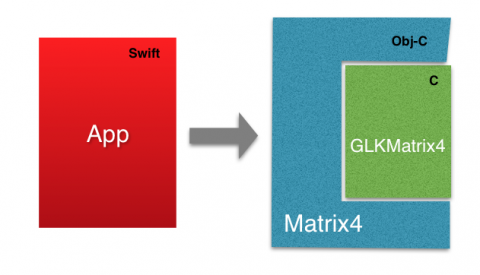Again, you’ll learn more about matrices later; this just gives you a quick overview of the class.

## Refactoring to a Node Class

For now, the starter project has everything set up in ViewController.swift. Although this was the easiest way to get started, it won’t scale well as your app gets larger.

In this section, you’ll refactor your project through the following five steps:

1. Creating a Vertex Structure
2. Creating a Node Class
3. Creating a Triangle Subclass

Time to get started!

Note: This section is optional, since at the end of this section you’ll be right back where you started, albeit with a cleaner project and a colored triangle. If you’d like go straight to the 3D stuff, feel free to skip ahead to the “Creating a Cube” section. A starter project will be waiting there for you.

### 1) Creating a Vertex Structure

Create a new class with the iOS\Source\Swift File template and name it Vertex.swift.

Open Vertex.swift and replace its contents with the following:

```struct Vertex{

var x,y,z: Float     // position data
var r,g,b,a: Float   // color data

func floatBuffer() -> [Float] {
return [x,y,z,r,g,b,a]
}

}
```

This is a structure to store the position and color of each vertex. `floatBuffer()` is a handy method that returns the vertex data as an array of Floats in strict order.

### 2) Create a Node Class

Create a new class with the iOS\Source\Swift File template and name it Node.swift.

Open Node.swift and replace the contents with the following:

```import Foundation
import Metal
import QuartzCore

class Node {

let device: MTLDevice
let name: String
var vertexCount: Int
var vertexBuffer: MTLBuffer

init(name: String, vertices: Array<Vertex>, device: MTLDevice){
// 1
var vertexData = Array<Float>()
for vertex in vertices{
vertexData += vertex.floatBuffer()
}

// 2
let dataSize = vertexData.count * MemoryLayout.size(ofValue: vertexData)
vertexBuffer = device.makeBuffer(bytes: vertexData, length: dataSize, options: [])

// 3
self.name = name
self.device = device
vertexCount = vertices.count
}

}
```

Going over this code section by section:

Since `Node` represents an object to draw, you need to provide it with the vertices it contains, a name for convenience, and a device to create buffers and render later on.

1. Go through each vertex and form a single buffer with floats, which will look like this:
2. Then, ask the device to create a vertex buffer with the float buffer you created above.
3. Finally, you set the instance variables.

Nothing fancy, eh?

Next, you need to move some of the render code that is currently in `ViewController` to `Node`. Specifically, you want to move the code responsible for rendering a particular buffer of vertices.

To do this, add this method to Node.swift:

```func render(commandQueue: MTLCommandQueue, pipelineState: MTLRenderPipelineState, drawable: CAMetalDrawable, clearColor: MTLClearColor?){

let renderPassDescriptor = MTLRenderPassDescriptor()
renderPassDescriptor.colorAttachments.texture = drawable.texture
renderPassDescriptor.colorAttachments.clearColor =
MTLClearColor(red: 0.0, green: 104.0/255.0, blue: 5.0/255.0, alpha: 1.0)
renderPassDescriptor.colorAttachments.storeAction = .store

let commandBuffer = commandQueue.makeCommandBuffer()

let renderEncoder = commandBuffer.makeRenderCommandEncoder(descriptor: renderPassDescriptor)
renderEncoder.setRenderPipelineState(pipelineState)
renderEncoder.setVertexBuffer(vertexBuffer, offset: 0, at: 0)
renderEncoder.drawPrimitives(type: .triangle, vertexStart: 0, vertexCount: vertexCount,
instanceCount: vertexCount/3)
renderEncoder.endEncoding()

commandBuffer.present(drawable)
commandBuffer.commit()
}
```

This code should be a review from the previous tutorial. You can see that it’s been copied from `ViewController`‘s `render()` method, but updated to use the vertex data for this node.

That’s it for the node class for now – time to create a subclass for the triangle!

### 3) Create a Triangle Subclass

Create a new class with the iOS\Source\Swift File template and name it Triangle.swift.

Open Triangle.swift and replace the contents with the following:

```import Foundation
import Metal

class Triangle: Node {

init(device: MTLDevice){

let V0 = Vertex(x:  0.0, y:   1.0, z:   0.0, r:  1.0, g:  0.0, b:  0.0, a:  1.0)
let V1 = Vertex(x: -1.0, y:  -1.0, z:   0.0, r:  0.0, g:  1.0, b:  0.0, a:  1.0)
let V2 = Vertex(x:  1.0, y:  -1.0, z:   0.0, r:  0.0, g:  0.0, b:  1.0, a:  1.0)

let verticesArray = [V0,V1,V2]
super.init(name: "Triangle", vertices: verticesArray, device: device)
}

}
```

Here you subclass the `Node` class you just wrote for the triangle. In the initializer, you define the three vertices that make up the triangle, and pass that data to the superclass’s initializer.

It’s looking nice and clean already!

### 4) Refactor your View Controller

Now that you have all of the pieces in place, let’s refactor your view controller to use your new `Triangle` class.

Open ViewController.swift and delete the following line:

```var vertexBuffer: MTLBuffer! = nil
```

The node object holds `vertexBuffer`, so you won’t need it here.

Next, replace:

```let vertexData:[Float] = [
0.0, 1.0, 0.0,
-1.0, -1.0, 0.0,
1.0, -1.0, 0.0]
```

with the following:

```var objectToDraw: Triangle!
```

And replace:

```// 1
let dataSize = vertexData.count * sizeofValue(vertexData)
// 2
vertexBuffer = device.newBufferWithBytes(vertexData, length: dataSize, options: nil)
```

with:

```objectToDraw = Triangle(device: device)
```

Now, when `objectToDraw` initializes and is ready to go, the only thing that’s missing a call to the draw method from `objectToDraw` in the ViewController render method.

Finally, replace the `render()` method with the following:

```func render() {
guard let drawable = metalLayer?.nextDrawable() else { return }
objectToDraw.render(commandQueue: commandQueue, pipelineState: pipelineState, drawable: drawable, clearColor: nil)
}
```

Build and run, and… uh-oh, where’d the triangle go?

Do you have any ideas why that might be? Try to think of an answer, and then check to see if you are correct. Hint: take a look at the data inside the `Vertex` structure.

[spoiler]`Vertex` now contains color components, but in your vertex shader, you only expect three components for x, y and z.[/spoiler]

Open Shaders.metal and take a good look at the vertex shader. You’ll notice it returns a `float4` value for the position of each vertex and takes an array of `packed_float3`, which is data from a vertex buffer.

Now you’ll create two structures to hold `Vertex` data that passes to vertex shader, and one for vertex shader to return. It’ll be more clear when you see the code.

Add this to `Shaders.metal` below `using namespace metal;` :

```struct VertexIn{
packed_float3 position;
packed_float4 color;
};

struct VertexOut{
float4 position [[position]];  //1
float4 color;
};
```

You want the vertex shader function to return `VertexOut` struct instead of just `float4`.

Note that vertex shaders must always return a position. In `VertexOut`, you specify the position component with special qualifier `[[position]]`.

Now, modify the vertex shader code, so it looks like the following:

```vertex VertexOut basic_vertex(                           // 1
const device VertexIn* vertex_array [[ buffer(0) ]],   // 2
unsigned int vid [[ vertex_id ]]) {

VertexIn VertexIn = vertex_array[vid];                 // 3

VertexOut VertexOut;
VertexOut.position = float4(VertexIn.position,1);
VertexOut.color = VertexIn.color;                       // 4

return VertexOut;
}
```

Taking each commented section in turn:

1. Mark the vertex shader as returning a `VertexOut` instead of a `float4`.
2. Mark the `vertex_array` as containing `VertexIn` vertices instead of `packed_float3`. Note that the `VertexIn` structure maps to the `Vertex` structure you created earlier.
3. Get the current vertex from the array.
4. Create a `VertexOut` and pass data from `VertexIn` to `VertexOut`, which returns at the end.

You might ask, “Why not to use `VertexIn` as a return value, since the data is the same?”

Good question! That would work at the moment, but you’d need different structures later after applying transformations to the position value.

Build and run. Oh, look who’s back!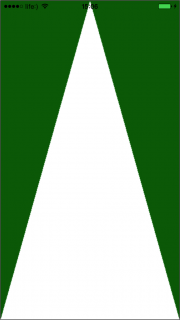Triangle’s back, tell a friend…

But you haven’t used the vertex color component, which now passes to the vertex shader. Fix that by modifying the fragment shader to look like this:

```fragment half4 basic_fragment(VertexOut interpolated [[stage_in]]) {  //1
return half4(interpolated.color, interpolated.color, interpolated.color, interpolated.color); //2
}
```

A short bit of code, but important all the same:

1. The vertex shader passes the `VertexOut` structure, but its values will be interpolated based on the position of the fragment you’re rendering. More on this later.
2. Now you simply return the color for the current fragment instead of the hardcoded white color.

Build and run. You should be blinded by colors: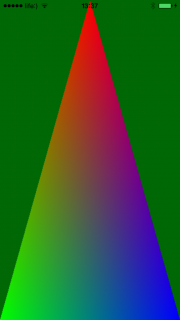You may be wondering how you got rainbow colors in the middle of the triangle, considering you only specified three color values in the triangle.

As hinted earlier, the color values are interpolated based on the fragment you’re drawing. For example, the fragment on the bottom of the triangle 50% between the green and blue vertices would be blended as 50% green and 50% blue. This is done for you automatically, for any kind of value that you pass from the vertex shader to the fragment shader.

Okay, very nicely done so far! Now that your project is refactored, it will be much easier to change from a triangle to a cube.

## Creating a Cube

Note: If you skipped the previous section, download this starter project with the newly refactored code. The only difference other than general cleanliness is that the triangle is now colored. Feel free to take a look through and get comfortable with the changes.

Your next task is to create a cube instead of a triangle. For that, as with all object models, you’ll create a subclass of the `Node` class.

Create a new class with the iOS\Source\Swift File template and name it Cube.swift.

Open Cube.swift and replace the contents with the following:

```import Foundation
import Metal

class Cube: Node {

init(device: MTLDevice){

let A = Vertex(x: -1.0, y:   1.0, z:   1.0, r:  1.0, g:  0.0, b:  0.0, a:  1.0)
let B = Vertex(x: -1.0, y:  -1.0, z:   1.0, r:  0.0, g:  1.0, b:  0.0, a:  1.0)
let C = Vertex(x:  1.0, y:  -1.0, z:   1.0, r:  0.0, g:  0.0, b:  1.0, a:  1.0)
let D = Vertex(x:  1.0, y:   1.0, z:   1.0, r:  0.1, g:  0.6, b:  0.4, a:  1.0)

let Q = Vertex(x: -1.0, y:   1.0, z:  -1.0, r:  1.0, g:  0.0, b:  0.0, a:  1.0)
let R = Vertex(x:  1.0, y:   1.0, z:  -1.0, r:  0.0, g:  1.0, b:  0.0, a:  1.0)
let S = Vertex(x: -1.0, y:  -1.0, z:  -1.0, r:  0.0, g:  0.0, b:  1.0, a:  1.0)
let T = Vertex(x:  1.0, y:  -1.0, z:  -1.0, r:  0.1, g:  0.6, b:  0.4, a:  1.0)

let verticesArray:Array<Vertex> = [
A,B,C ,A,C,D,   //Front
R,T,S ,Q,R,S,   //Back

Q,S,B ,Q,B,A,   //Left
D,C,T ,D,T,R,   //Right

Q,A,D ,Q,D,R,   //Top
B,S,T ,B,T,C    //Bot
]

super.init(name: "Cube", vertices: verticesArray, device: device)
}
}
```

That looks rather familiar, don’t you think? It’s almost a carbon copy of the Triangle implementation: it just has eight vertices instead of three.

Also, each side comprises two triangles. For a better understanding, it might help to sketch it out on paper.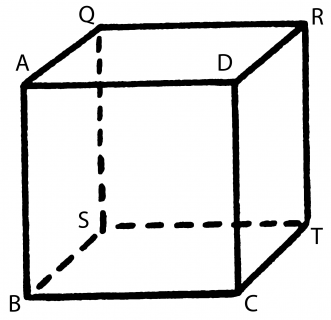Next open ViewController.swift and change the `objectToDraw` property to a cube:

```var objectToDraw: Cube!
```

Inside `init()`, change the line that initializes `objectToDraw` to make it a cube:

```objectToDraw = Cube(device: device)
```

Build and run. It might not look like it, but believe it or not, you’ve created a cube!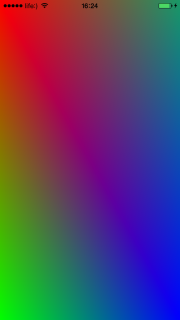What you see now is just the cube’s front face — an up-close selfie, if you will. It’s also stretched over the display aspect ratio.

Don’t believe me? Doubt your cube-making skills? Okay, for your own sanity you’ll modify `Cube` so it’s smaller.

Rework the lines with vertices data so they look like this:

```let A = Vertex(x: -0.3, y:   0.3, z:   0.3, r:  1.0, g:  0.0, b:  0.0, a:  1.0)
let B = Vertex(x: -0.3, y:  -0.3, z:   0.3, r:  0.0, g:  1.0, b:  0.0, a:  1.0)
let C = Vertex(x:  0.3, y:  -0.3, z:   0.3, r:  0.0, g:  0.0, b:  1.0, a:  1.0)
let D = Vertex(x:  0.3, y:   0.3, z:   0.3, r:  0.1, g:  0.6, b:  0.4, a:  1.0)

let Q = Vertex(x: -0.3, y:   0.3, z:  -0.3, r:  1.0, g:  0.0, b:  0.0, a:  1.0)
let R = Vertex(x:  0.3, y:   0.3, z:  -0.3, r:  0.0, g:  1.0, b:  0.0, a:  1.0)
let S = Vertex(x: -0.3, y:  -0.3, z:  -0.3, r:  0.0, g:  0.0, b:  1.0, a:  1.0)
let T = Vertex(x:  0.3, y:  -0.3, z:  -0.3, r:  0.1, g:  0.6, b:  0.4, a:  1.0)
```

Build and run.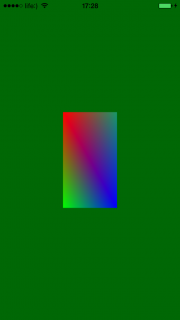The cube is smaller, but something just doesn’t feel right here. Wait — it’s probably that you have to painstakingly modify vertices every time you need to modify a node. There’s got to be a better way!

And indeed there is. This is where matrices come in.

## Introduction to Matrices

First things first: what is a matrix?

A matrix is a rectangular array of numbers (sorry, Neo!). In 3D gaming, you’ll often see a 4×4 sized matrix, with four columns and four rows.

Also note that you’re using the GLKit column-based GLKMatrix4, so elements are placed like this: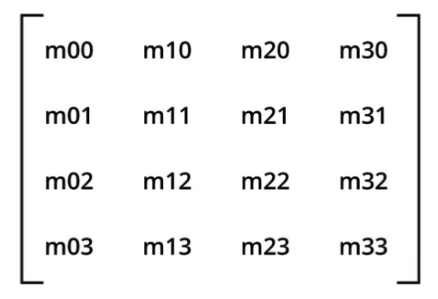With matrices, you can transform your object in three ways:

1. Translation: Shift the object along the x, y and z axis.
2. Rotation: Rotate the object around any axis.
3. Scale: Change the object size along any axis. In this tutorial, you’ll always scale proportionally along all axes.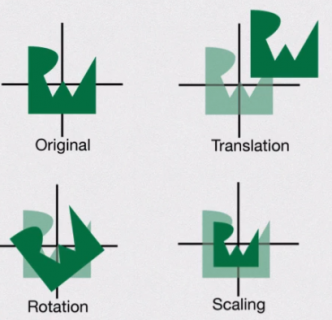How does this work? First, you create an instance of `Matrix4`, like this (you don’t need to add this or the rest of the code in this section, this is just to illustrate):

```var modelTransformationMatrix = Matrix4()
```

Then you use apply transformations, like this:

```modelTransformationMatrix.translate(positionX, y: positionY, z: positionZ)
modelTransformationMatrix.rotateAroundX(rotationX, y: rotationY, z: rotationZ)
modelTransformationMatrix.scale(scale, y: scale, z: scale)
```

There’s some math behind this that you can learn about in any Linear Algebra course. It’s great if you understand it, but it’s not necessary for this tutorial.

Before you continue, open HelloMetal-BridgingHeader.h and import your `Matrix4` class. You’ll need that in the following sections.

```#import "Matrix4.h"
```

## Model Transformation

The first transformation you’ll need is the model transformation. This transformation converts your node’s coordinates from local coordinates to world coordinates. In other words, it lets you move your model around your world.

Let’s see how this works. Open Node.swift and add the following new properties:

```var positionX: Float = 0.0
var positionY: Float = 0.0
var positionZ: Float = 0.0

var rotationX: Float = 0.0
var rotationY: Float = 0.0
var rotationZ: Float = 0.0
var scale: Float     = 1.0
```

These are convenience properties that you will set in order to position, rotate, or scale the node within the world. You will construct a model matrix from these which you will use to apply the model transformation.

To do this, add the following method at the end of `Node`:

```func modelMatrix() -> Matrix4 {
let matrix = Matrix4()
matrix.translate(positionX, y: positionY, z: positionZ)
matrix.rotateAroundX(rotationX, y: rotationY, z: rotationZ)
matrix.scale(scale, y: scale, z: scale)
return matrix
}
```

In this method, you generate a matrix from those parameters.

Now you need to pass this matrix to the shader so it can apply the model transformation. To do this, you need to understand the concept of uniform data.

## Uniform Data

So far, you have passed different data for each vertex to the shaders through vertex arrays. But the model matrix will be the same across an entire model, so it would be a waste of space to copy it for each vertex.

When you have identical data across an entire model, you can instead pass the data to the shader as uniform data.

The first step to do this is to put your data into a buffer object, which represents memory accessible to both the CPU and the GPU.

In Node.swift, add this right after `renderEncoder.setVertexBuffer(self.vertexBuffer, offset: 0, atIndex: 0)`:

```// 1
let nodeModelMatrix = self.modelMatrix()
// 2
let uniformBuffer = device.makeBuffer(length: MemoryLayout<Float>.size * Matrix4.numberOfElements(), options: [])
// 3
let bufferPointer = uniformBuffer.contents()
// 4
memcpy(bufferPointer, nodeModelMatrix.raw(), MemoryLayout<Float>.size * Matrix4.numberOfElements())
// 5
renderEncoder.setVertexBuffer(uniformBuffer, offset: 0, at: 1)
```

Here’s the section-by-section breakdown:

1. Call the method you wrote earlier to convert the convenience properties (like position and rotation) into a model matrix.
2. Ask the device to create a buffer with shared CPU/GPU memory.
3. Get a raw pointer from buffer (similar to `void *` in Objective-C).
4. Copy your matrix data into the buffer.
5. Pass `uniformBuffer` (with data copied) to the vertex shader. This is similar to how you sent the buffer of vertex-specific data, except you use index 1 instead of 0.

There is one problem with this code: it’s in a render method that should be called, hopefully, 60 times per second. This means you’re creating a new buffer 60 times per second.

Continuously allocating memory each frame is expensive and not recommended in production apps; you’ll learn a better way to do this in future tutorials but this approach will do for now. You can see how this is done in the iOS Metal Game template.

You’ve passed the matrix to the vertex shader, but you’re not using it yet. To fix this, open Shaders.metal and add this structure right below `VertexOut`:

```struct Uniforms{
float4x4 modelMatrix;
};
```

Right now this only holds one component, but later you’ll use it to hold one more matrix.

```vertex VertexOut basic_vertex(
const device VertexIn* vertex_array [[ buffer(0) ]],
const device Uniforms&  uniforms    [[ buffer(1) ]],           //1
unsigned int vid [[ vertex_id ]]) {

float4x4 mv_Matrix = uniforms.modelMatrix;                     //2

VertexIn VertexIn = vertex_array[vid];

VertexOut VertexOut;
VertexOut.position = mv_Matrix * float4(VertexIn.position,1);  //3
VertexOut.color = VertexIn.color;

return VertexOut;
}
```

Here’s what’s going on with this chunk of code:

1. You add a second parameter for the uniform buffer, marking that it’s incoming in slot 1 to match up with the code you wrote earlier.
2. You then get a handle to the model matrix in the uniforms structure.
3. To apply the model transformation to a vertex, you simply multiply the vertex position by the model matrix.

You’re done with that part; now back to test the cube.

In `Cube.swift`, change the vertices back to this:

```let A = Vertex(x: -1.0, y:   1.0, z:   1.0, r:  1.0, g:  0.0, b:  0.0, a:  1.0)
let B = Vertex(x: -1.0, y:  -1.0, z:   1.0, r:  0.0, g:  1.0, b:  0.0, a:  1.0)
let C = Vertex(x:  1.0, y:  -1.0, z:   1.0, r:  0.0, g:  0.0, b:  1.0, a:  1.0)
let D = Vertex(x:  1.0, y:   1.0, z:   1.0, r:  0.1, g:  0.6, b:  0.4, a:  1.0)

let Q = Vertex(x: -1.0, y:   1.0, z:  -1.0, r:  1.0, g:  0.0, b:  0.0, a:  1.0)
let R = Vertex(x:  1.0, y:   1.0, z:  -1.0, r:  0.0, g:  1.0, b:  0.0, a:  1.0)
let S = Vertex(x: -1.0, y:  -1.0, z:  -1.0, r:  0.0, g:  0.0, b:  1.0, a:  1.0)
let T = Vertex(x:  1.0, y:  -1.0, z:  -1.0, r:  0.1, g:  0.6, b:  0.4, a:  1.0)
```

In `ViewController.swift`, add the following after `objectToDraw = Cube(device: device)`:

```objectToDraw.positionX = -0.25
objectToDraw.scale = 0.5
```

Build and run; as expected, the cube has been scaled down, shifted left and rotated 45 degrees around the Z axis.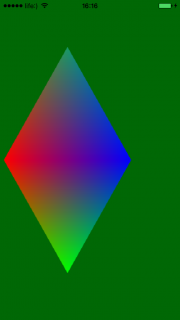This proves one thing: mathematical matrices are much cooler than any other matrices! :]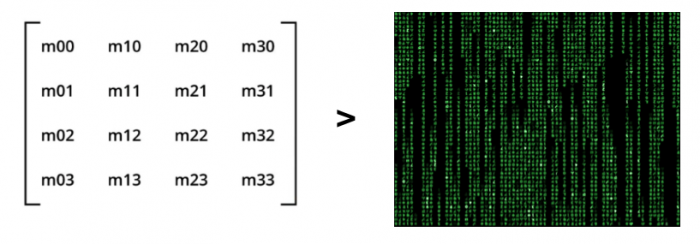Now it’s time for some science. You’ll shift the cube along the X , Y and Z axes all at once. For that, add following code right below `objectToDraw.positionX = -0.25`:

```objectToDraw.positionY =  0.25
objectToDraw.positionZ = -0.25
```

Build and run. The cube shifts along X and Y, but what’s wrong with Z?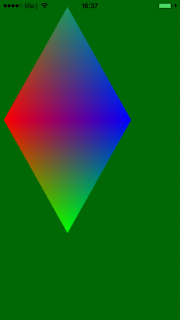You set `.positionZ` to be `-0.25`, so the cube should have moved away from you, but it didn’t. You might think there’s some problem with the matrix, but that’s not the problem at all. In fact, the cube did move back, but you can’t see it.

To understand the problem, you need to understand another type of transform: the projection transform.

## Projection Transformation

A projection transformation converts your node’s coordinates from camera coordinates to normalized coordinates. Depending on the type of projection you use, you’ll get different effects.

There are two projection types to understand here: orthographic and perspective.

in the image beow, the perspective projection is on the left, and the orthographic projection is on the right. The camera, or point of view, is located on the axis origin.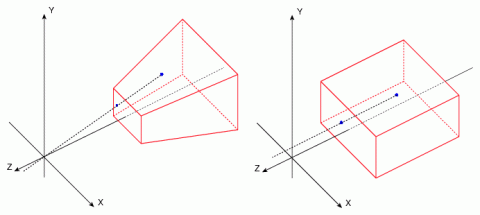Understanding perspective projection is easy, because it’s similar to how your eyes see the world. Orthographic is a bit harder, but easier than you might think, as you’ve been working with the cube in orthographic mode all along!

Look at it another way: imagine you’re standing on a railway and looking down the tracks. In perspective mode, the rails would look like this:In orthographic mode, the picture would be deformed and the rails parallel.

In the picture below, you can see another perspective projection. It’s a chopped pyramid, and inside that pyramid is where your scene renders. The whole scene is projected onto the pyramid’s top face, which represents your device’s screen: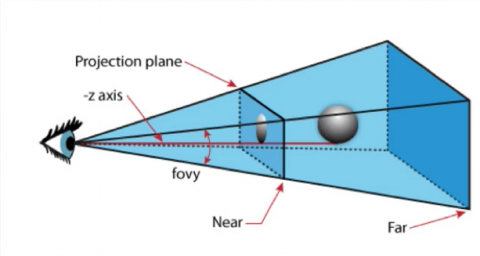Right now, Metal renders everything using orthographic projection, so you need to transform the scene to a perspective appearance. This calls for a matrix that describes perspective projection.

To recap the whole concept, you have a cube (your scene space), and you want to transform it into a chopped pyramid. To do that, you’ll create a projection matrix that describes the chopped pyramid above and maps it to your normalized box.

`Matrix4` already has a method to create a perspective projection matrix, so you’ll work with that first.

Add the following new property to ViewController.swift:

```var projectionMatrix: Matrix4!
```

Next, add the following to the top of `viewDidLoad()`:

```projectionMatrix = Matrix4.makePerspectiveViewAngle(Matrix4.degrees(toRad: 85.0), aspectRatio: Float(self.view.bounds.size.width / self.view.bounds.size.height), nearZ: 0.01, farZ: 100.0)
```

85.0 degrees specifies the camera’s vertical angle of view. You don’t need a horizontal value because you pass the aspect ratio of the view along with near and far planes to specify the field of view.

In other words, everything that is too close or too far away from the camera won’t be displayed.

Now, modify `render()` inside Node.swift to add another parameter:

```func render(commandQueue: MTLCommandQueue, pipelineState: MTLRenderPipelineState, drawable: CAMetalDrawable, projectionMatrix: Matrix4, clearColor: MTLClearColor?) {
```

Now you want to include `projectionMatrix` in the uniform buffer, which you’ll pass to the vertex shader later. Since the uniform buffer will now contain two matrices instead of one, you’ll need to increase its size.

Find the following:

```let uniformBuffer = device.makeBuffer(length: MemoryLayout<Float>.size * Matrix4.numberOfElements(), options: [])
```

…and replace it with this:

```let uniformBuffer = device.makeBuffer(length: MemoryLayout<Float>.size * Matrix4.numberOfElements() * 2, options: [])
```

Now find the following:

```memcpy(bufferPointer, nodeModelMatrix.raw(), MemoryLayout<Float>.size*Matrix4.numberOfElements())
```

And add this line right afterwards:

```memcpy(bufferPointer + MemoryLayout<Float>.size * Matrix4.numberOfElements(), projectionMatrix.raw(), MemoryLayout<Float>.size * Matrix4.numberOfElements())
```

Now both matrices are passed in to the uniforms buffer. All you need to do now is use this projection matrix in your shader.

Go to Shaders.metal and modify `Uniforms` to include `projectionMatrix`:

```struct Uniforms{
float4x4 modelMatrix;
float4x4 projectionMatrix;
};
```

You now need to get the projection matrix. Find the following in the vertex shader:

```float4x4 mv_Matrix = uniforms.modelMatrix;
```

And add this just after the above line:

```float4x4 proj_Matrix = uniforms.projectionMatrix;
```

To apply this matrix transformation to your position, you simply need to multiply the matrix and the position, just as you did with `modelMatrix`.

To that end, replace:

```VertexOut.position = mv_Matrix * float4(VertexIn.position,1);
```

with the following:

```VertexOut.position = proj_Matrix * mv_Matrix * float4(VertexIn.position,1);
```

Finally, you need to pass in the projection matrix from your render method in ViewController.swift. Replace the following:

```objectToDraw.render(commandQueue, pipelineState: pipelineState, drawable: drawable, clearColor: nil)
```

With this:

```objectToDraw.render(commandQueue: commandQueue, pipelineState: pipelineState, drawable: drawable,projectionMatrix: projectionMatrix, clearColor: nil)
```

Now change the translation parameters for `objectToDraw` so it looks like this:

```objectToDraw.positionX = 0.0
objectToDraw.positionY =  0.0
objectToDraw.positionZ = -2.0
objectToDraw.scale = 0.5
```

Build and run; it looks somewhat like a cube, but it’s still messed up: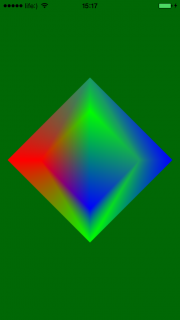You’ll fix this in a moment, just after a quick recap of what you’ve just accomplished:

1. You added a model transformation, which allows you to modify an object’s location, size and rotation.
2. You added a projection transformation, which allows you to shift from an orthographic to a more natural perspective projection.

There are actually two more transformations beyond this that are typically used in a 3D rendering pipeline:

1. View transformation: What if you want to look at the scene from a different position? You could move every object in the scene by modifying all of their model transformations, but this is inefficient. It’s often convenient to have a separate transformation that represents how you’re looking at the scene, which is your “camera”.
2. Viewport transformation: This takes the little world you’ve created in normalized coordinates and maps it to the device screen. This is handled automatically by Metal, so you don’t need to do anything; it’s just something worth knowing.

Here’s the plan for the rest of the tutorial:

2. Make the cube rotate.
3. Fix your cube’s peculiar transparency.

## View Transformation

A view transformation converts your node’s coordinates from world coordinates to camera coordinates. In other words, it allows you to move your camera around your world.

Adding a view transformation is fairly easy. Open Node.swift and change the render method declaration so it looks like this:

```func render(commandQueue: MTLCommandQueue, pipelineState: MTLRenderPipelineState, drawable: CAMetalDrawable, parentModelViewMatrix: Matrix4, projectionMatrix: Matrix4, clearColor: MTLClearColor?) {
```

`parentModelViewMatrix`, which represents the camera position, will be put to use to transform the scene.

Inside `render()`, find:

```let nodeModelMatrix = self.modelMatrix()
```

```nodeModelMatrix.multiplyLeft(parentModelViewMatrix)
```

Note that you don’t pass this matrix to the shader as you did with the previous two matrices. Instead, you’re making a model view matrix which is a multiplication of the model matrix with the view matrix. It’s quite common to pass them pre-multiplied like this for efficiency.

Now, you just need to pass the new parameter to `render()`. Open ViewController.swift, and find the following:

```objectToDraw.render(commandQueue: commandQueue, pipelineState: pipelineState, drawable: drawable,projectionMatrix: projectionMatrix, clearColor: nil)
```

Change it to this:

```let worldModelMatrix = Matrix4()
worldModelMatrix.translate(0.0, y: 0.0, z: -7.0)

objectToDraw.render(commandQueue: commandQueue, pipelineState: pipelineState, drawable: drawable, parentModelViewMatrix: worldModelMatrix, projectionMatrix: projectionMatrix ,clearColor: nil)
```

Also, delete these lines:

```objectToDraw.positionX = 0.0
objectToDraw.positionY =  0.0
objectToDraw.positionZ = -2.0
objectToDraw.scale = 0.5
```

You don’t need to move the object back, because you’re moving the point of view instead.

Build and run to see what you have now: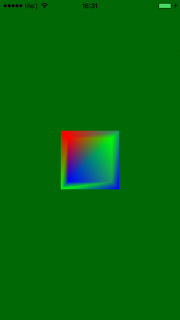To understand the View transformation a little better, you’ll make a few modifications.

Still in `render()`, find the following inside ViewController.swift:

```worldModelMatrix.translate(0.0, y: 0.0, z: -7.0)
```

And add this below the line above:

```worldModelMatrix.rotateAroundX(Matrix4.degrees(toRad: 25), y: 0.0, z: 0.0)
```

Build and run to see the effect of your modifications: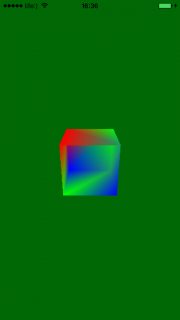You rotated the whole scene around the X-axis — or changed the camera direction, whichever way you prefer to think about it! :]

## A Rotating Cube

Now you’ll modify the project so it rotates your cube over time.

To do this, open Node.swift and add the following new property:

```var time:CFTimeInterval = 0.0
```

This is simply a property that tracks how long the node has been around.

Now, add the following method to the end of the class:

```func updateWithDelta(delta: CFTimeInterval){
time += delta
}
```

Next open ViewController.swift and add this new property:

```var lastFrameTimestamp: CFTimeInterval = 0.0
```

Next, change the following line:

```timer = CADisplayLink(target: self, selector: #selector(ViewController.gameloop))
```

To this:

```timer = CADisplayLink(target: self, selector: #selector(ViewController.newFrame(displayLink:)))
```

And replace this:

```func gameloop() {
autoreleasepool {
self.render()
}
}
```

With this:

```// 1

if lastFrameTimestamp == 0.0
{
}

// 2
let elapsed: CFTimeInterval = displayLink.timestamp - lastFrameTimestamp

// 3
gameloop(timeSinceLastUpdate: elapsed)
}

func gameloop(timeSinceLastUpdate: CFTimeInterval) {

// 4
objectToDraw.updateWithDelta(delta: timeSinceLastUpdate)

// 5
autoreleasepool {
self.render()
}
}
```

Nice work! Here’s what you did, step-by-step:

1. The display link now calls `newFrame()` every time the display refreshes. Note that the display link is passed as a parameter.
2. You calculate time between this frame and the previous one. Note that it’s inconsistent because some frames might be skipped.
3. You call `gameloop()` with the time interval since the last update.
4. You update your node by using `updateWithDelta()` before rendering.
5. Now, when a node is updated, you can call the render method.

Finally, you need to override `updateWithDelta` in `Cube` class. Open Cube.swift and add this new method:

```override func updateWithDelta(delta: CFTimeInterval) {

super.updateWithDelta(delta: delta)

let secsPerMove: Float = 6.0
rotationY = sinf( Float(time) * 2.0 * Float(M_PI) / secsPerMove)
rotationX = sinf( Float(time) * 2.0 * Float(M_PI) / secsPerMove)
}
```

Nothing fancy here; all you’re doing is calling `super()` to update the time property. Then you set the cube rotation properties to be a function of `sin`, which basically means that your cube will rotate to a point and then rotate back.

Build and run, and enjoy your rotating cube!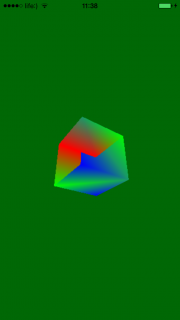Your cube should now have a little life to it, spinning back and forth like a little cube-shaped dancer.

## Fixing the Transparency

The last part is to fix the cube’s transparency — but first, you should understand the cause of the issue. It’s really quite simple and a little peculiar: Metal sometimes draws pixels of the back face of the cube before the front face.

So, how do you fix it?

There are two ways to fix this:

1. One approach is depth testing. With this method, you store each point’s depth value, so that when the two points are drawn at the same point on the screen, only the one with the lower depth is drawn.
2. The second approach is backface culling. This means that every triangle drawn is visible from only one side. In effect, the back face isn’t drawn until it turns toward the camera. This is based on the order you specify the vertices of the triangles.

You’re going to use backface culling to solve the problem here, as it’s a more efficient solution when there is only one model. The rule you must keep is this: all triangles must be drawn counter-clockwise, otherwise, they won’t be rendered.

Lucky for you, the cube vertices have been set up so every triangle is indeed specified as counter-clockwise, so you can just focus on learning how to use backface culling.

Open Node.swift and add find this in `render()`:

```let renderEncoder = commandBuffer.makeRenderCommandEncoder(descriptor: renderPassDescriptor)
```

```//For now cull mode is used instead of depth buffer
renderEncoder.setCullMode(MTLCullMode.front)
```

Build and run to see how your transparency issue looks: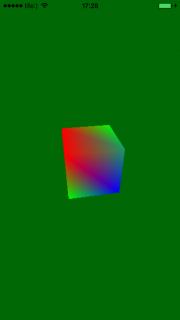Now your cube should be free of transparency. What a beautiful cube!

## Where to Go From Here?

Here is the final example project from this iOS Metal Tutorial.

Congratulations, you have learned a ton about moving to 3D in the new Metal API! You now have an understanding of model, view, projection, and viewport transformations, matrices, passing uniform data, backface culling, and more.

In the meantime, be sure to check out some great resources from Apple:

You also might enjoy the Beginning Metal course on our site, where we explain these same concepts in video form, but with even more detail.